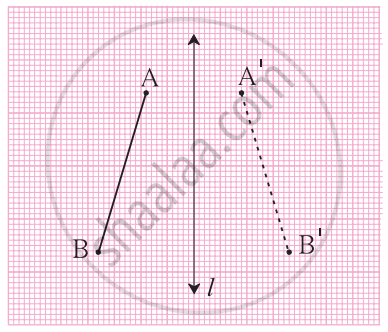# Drawing Symmetrical figures on graph paper:1. Draw a line segment AB and line l.

2. Now, measure the perpendicular distance of point A from the line l and take a point A’ at an equal distance from the opposite side of line l.

3. Now, measure the perpendicular distance of point B from the line l and take a point B’ at an equal distance from the opposite side of line l.

4. Joint point A’B’ as an equal reflection of line segment AB.

If you would like to contribute notes or other learning material, please submit them using the button below.

### Shaalaa.com

How to draw a symmetrical figure on a graph paper? [00:00:43]
S
0%